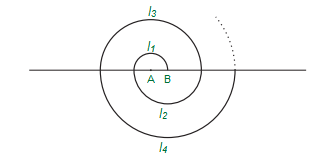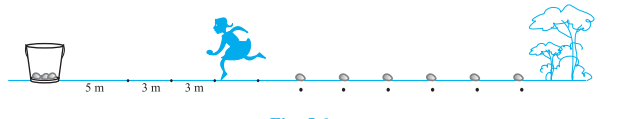# Class 10 NCERT Solutions – Chapter 5 Arithmetic Progressions – Exercise 5.3 | Set 2

• Last Updated : 28 May, 2021

### Question 11. If the sum of the first n terms of an AP is 4n − n2, what is the first term (that is S1)? What is the sum of first two terms? What is the second term? Similarly find the 3rd, the 10th and the nth terms.

Solution:

Given:

Sn = 4n−n2

First term can be obtained by putting n=1,

a = S1 = 4(1) − (1)2 = 4−1 = 3

Also,

Sum of first two terms = S2= 4(2)−(2)2 = 8−4 = 4

Second term, a2 = S2 − S1 = 4−3 = 1

Common difference, d = a2−a1 = 1−3 = −2

Also,

Nth term, an = a+(n−1)d

= 3+(n −1)(−2)

= 3−2n +2

= 5−2n

Therefore, a3 = 5−2(3) = 5-6 = −1

a10 = 5−2(10) = 5−20 = −15

Therefore, the sum of first two terms is equivalent to 4. The second term is 1.

And, the 3rd, the 10th, and the nth terms are −1, −15, and 5 − 2n respectively.

### Question 12. Find the sum of first 40 positive integers divisible by 6.

Solution:

The first positive integers that are divisible by 6 are 6, 12, 18, 24 ….

Noticing this series,

First term, a = 6

Common difference, d= 6.

Sum of n terms, we know,

Sn = n/2 [2a +(n – 1)d]

Substituting the values, we get

S40 = 40/2 [2(6)+(40-1)6]

= 20[12+(39)(6)]

= 20(12+234)

= 20×246

= 4920

### Question 13. Find the sum of first 15 multiples of 8.

Solution:

The first few multiples of 8 are 8, 16, 24, 32…

Noticing this series,

First term, a = 8

Common difference, d = 8.

Sum of n terms, we know,

Sn = n/2 [2a+(n-1)d]

Substituting the values, we get,

S15 = 15/2 [2(8) + (15-1)8]

= 15/2[6 +(14)(8)]

= 15/2[16 +112]

= 15(128)/2

= 15 × 64

= 960

### Question 14. Find the sum of the odd numbers between 0 and 50.

Solution:

The odd numbers between 0 and 50 are 1, 3, 5, 7, 9 … 49.

Therefore, we can see that these odd numbers are in the form of A.P.

Now,

First term, a = 1

Common difference, d = 2

Last term, l = 49

Last term is equivalent to,

l = a+(n−1) d

49 = 1+(n−1)2

48 = 2(n − 1)

n − 1 = 24

Solving for n, we get,

n = 25

Sum of nth term,

Sn = n/2(a +l)

Substituting these values,

S25 = 25/2 (1+49)

= 25(50)/2

=(25)(25)

= 625

### Question 15. A contract on construction job specifies a penalty for delay of completion beyond a certain date as follows: Rs. 200 for the first day, Rs. 250 for the second day, Rs. 300 for the third day, etc., the penalty for each succeeding day being Rs. 50 more than for the preceding day. How much money the contractor has to pay as penalty, if he has delayed the work by 30 days.

Solution:

The given penalties form and A.P. having first term, a = 200 and common difference, d = 50.

By the given constraints,

Penalty that has to be paid if contractor has delayed the work by 30 days = S30

Sum of nth term, we know,

Sn = n/2[2a+(n -1)d]

Calculating, we get,

S30= 30/2[2(200)+(30 – 1)50]

= 15[400+1450]

= 15(1850)

= 27750

Therefore, the contractor has to pay Rs 27750 as penalty for 30 days delay.

### Question 16. A sum of Rs 700 is to be used to give seven cash prizes to students of a school for their overall academic performance. If each prize is Rs 20 less than its preceding prize, find the value of each of the prizes.

Solution:

Let us assume the cost of 1st prize be Rs. P.

Then, cost of 2nd prize = Rs. P − 20

Also, cost of 3rd prize = Rs. P − 40

These prizes form an A.P., with common difference, d = −20 and first term, a = P.

Given that, S7 = 700

Sum of nth term,

Sn = n/2 [2a + (n – 1)d]

Substituting these values, we get,

7/2 [2a + (7 – 1)d] = 700

Solving, we get,

a + 3(−20) = 100

a −60 = 100

a = 160

Therefore, the value of each of the prizes was Rs 160, Rs 140, Rs 120, Rs 100, Rs 80, Rs 60, and Rs 40.

### Question 17. In a school, students thought of planting trees in and around the school to reduce air pollution. It was decided that the number of trees, that each section of each class will plant, will be the same as the class, in which they are studying, e.g., a section of class I will plant 1 tree, a section of class II will plant 2 trees and so on till class XII. There are three sections of each class. How many trees will be planted by the students?

Solution:

The number of trees planted by the students form an AP, 1, 2, 3, 4, 5………………..12

Now,

First term, a = 1

Common difference, d = 2−1 = 1

Sum of nth term,

Sn = n/2 [2a +(n-1)d]

S12 = 12/2 [2(1)+(12-1)(1)]

= 6(2+11)

= 6(13)

= 78

Number of trees planted by 1 section of the classes = 78

Therefore,

Number of trees planted by 3 sections of the classes = 3×78 = 234

### Question 18. A spiral is made up of successive semicircles, with centres alternately at A and B, starting with centre at A of radii 0.5, 1.0 cm, 1.5 cm, 2.0 cm, ……… as shown in figure. What is the total length of such a spiral made up of thirteen consecutive semicircles? (Take π = 22/7)Solution:

We know,

Perimeter of a semi-circle = πr

Calculating ,

P1 = π(0.5) = π/2 cm

P2 = π(1) = π cm

P3 = π(1.5) = 3π/2 cm

Where, P1, P2, P3 are the lengths of the semi-circles respectively.

Now, this forms a series, such that,

π/2, π, 3π/2, 2π, ….

P1 = π/2 cm

P2 = π cm

Common difference, d = P2 – P1 = π – π/2 = π/2

First term = P1= a = π/2 cm

Sum of nth term,

Sn = n/2 [2a + (n – 1)d]

Therefore, Sum of the length of 13 consecutive circles is;

S13 = 13/2 [2(π/2) + (13 – 1)π/2]

Solving, we get,

=  13/2 [π + 6π]

=13/2 (7π)

= 13/2 × 7 × 22/7

= 143 cm

### Question 19. 200 logs are stacked in the following manner: 20 logs in the bottom row, 19 in the next row, 18 in the row next to it and so on. In how many rows are the 200 logs placed and how many logs are in the top row?Solution:

The numbers of logs in rows are in the form of an A.P. 20, 19, 18…

Given,

First term, a = 20 and common difference, d = a2−a1 = 19−20 = −1

Let us assume a total of 200 logs to be placed in n rows.

Thus, Sn = 200

Sum of nth term,

Sn = n/2 [2a +(n -1)d]

S12 = 12/2 [2(20)+(n -1)(-1)]

400 = n (40−n+1)

400 = n (41-n)

400 = 41n−n2

Solving the eq, we get,

n2−41n + 400 = 0

n2−16n−25n+400 = 0

n(n −16)−25(n −16) = 0

(n −16)(n −25) = 0

Now,

Either (n −16) = 0 or n−25 = 0

n = 16 or n = 25

By the nth term formula,

an = a+(n−1)d

a16 = 20+(16−1)(−1)

= 20−15

= 5

And, the 25th term is,

a25 = 20+(25−1)(−1)

= 20−24

= −4

Therefore, 200 logs can be placed in 16 rows and the number of logs in the 16th row is 5, since the number of logs can’t be negative as in case of 25th term.

### Question 20. In a potato race, a bucket is placed at the starting point, which is 5 m from the first potato and other potatoes are placed 3 m apart in a straight line. There are ten potatoes in the line.### A competitor starts from the bucket, picks up the nearest potato, runs back with it, drops it in the bucket, runs back to pick up the next potato, runs to the bucket to drop it in, and she continues in the same way until all the potatoes are in the bucket. What is the total distance the competitor has to run?

[Hint: to pick up the first potato and the second potato, the total distance (in meters) run by a competitor is 2×5+2×(5+3)]

Solution:

The distances of potatoes from the bucket are 5, 8, 11, 14…, which form an AP.

Now, we know that  the distance run by the competitor for collecting these potatoes are two times of the distance at which the potatoes have been kept.

Therefore, distances to be run w.r.t distances of potatoes is equivalent to,

10, 16, 22, 28, 34,……….

We get, a = 10 and d = 16−10 = 6

Sum of nth term, we get,

S10 = 12/2 [2(20)+(n -1)(-1)]

= 5[20+54]

= 5(74)

Solving we get,

= 370

Therefore, the competitor will run a total distance of 370 m.

My Personal Notes arrow_drop_up